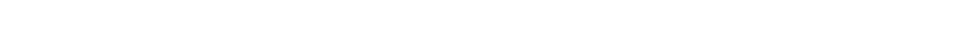Colloquium # 299

Can ergodicity impede statistical equilibration, and non-ergodicity support it?

By  Kushal K. Shah,
Associate Professor and Head, EECS Dept
Coordinator, Data Science & Engineering
Indian Institute of Science Education & Research [IISER] Bhopal

Friday, Dec 20th 2019 at 3:30 PM
Seminar HallAbstract

Equilibration of statistical systems is an experimentally observed fact, but is not very well understood from a dynamical perspective. An important dynamical model used to study this equilibration process is a particle of mass, m, moving along straight lines within a springy billiard and undergoing elastic collisions at the boundaries. It is known that if such springy billiards are ergodic, adiabatic invariants can prevent equilibration of these systems, especially when the mass of the particle, m, is much smaller than the mass of the moving billiard wall, M [1,2]. However, counter intuitively, we have shown that if a springy billiard is non-ergodic (i.e. has multiple ergodic components), then its equilibration rate can remain non-zero even in the limit of vanishing mass ratio (m/M tends to zero) . Interestingly, it turns out that in the case of ergodic billiards, if the time of ergodization in the frozen system is much longer than the time of equilibration, then also the system can still equilibrate over a finite time-scale for vanishing mass ratio .

 Neishtadt A. I. and Sinai Y. G. (2004) Adiabatic piston as a dynamical system. J. Stat. Phys. 116:815

 Wright P. (2007) The periodic oscillation of an adiabatic piston in two or three dimensions. Commun. Math. Phys. 275:553

 Shah K., Turaev D., Gelfreich V. and Rom-Kedar V. (2017) Equilibration of energy in slow-fast systems. Proc. Natl. Acad. Sci. USA 114:E10514

 Shah K. (2019) Smooth phase transition of energy equilibration in a springy Sinai billiard. Physical Review E 99:062204